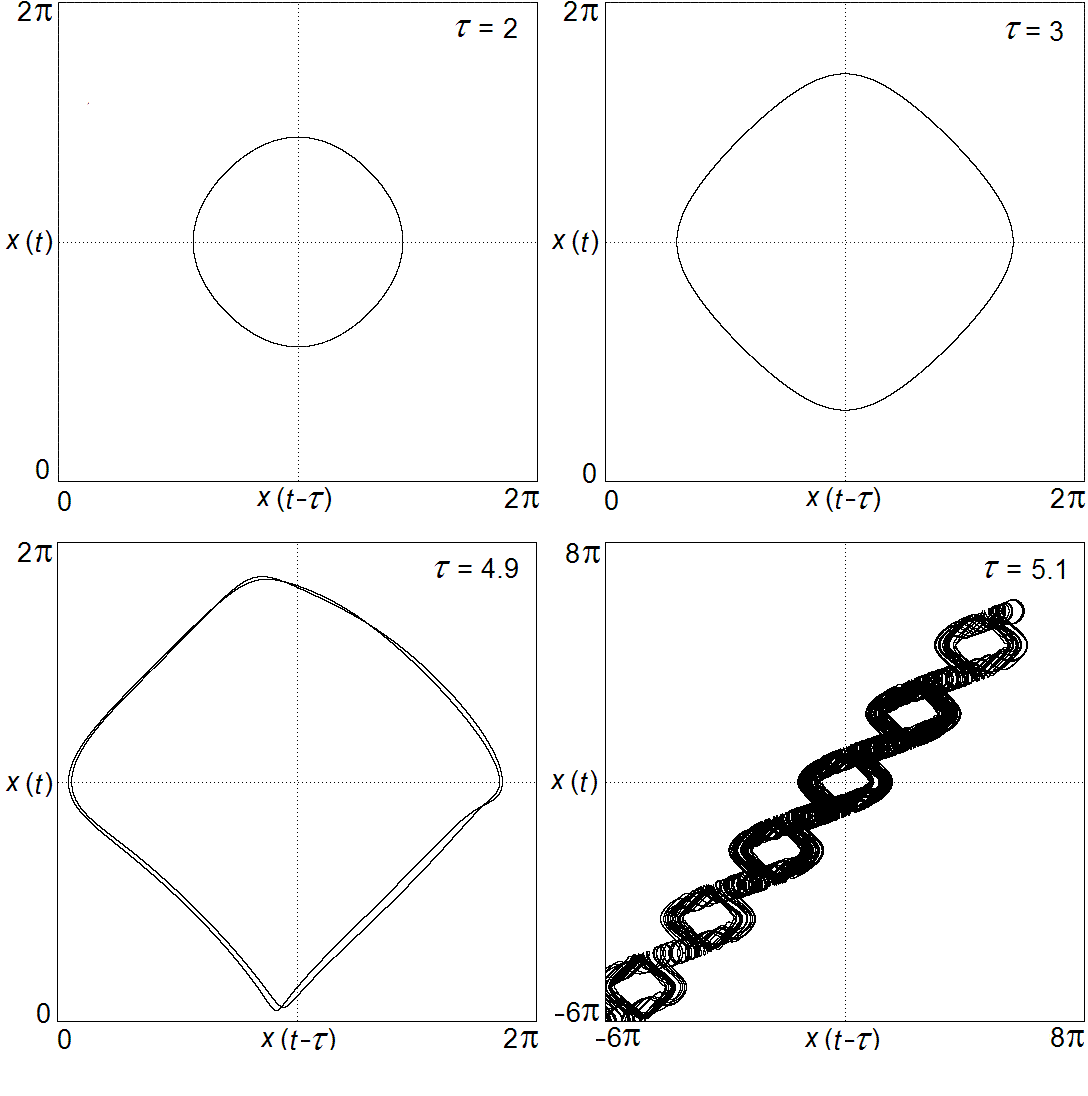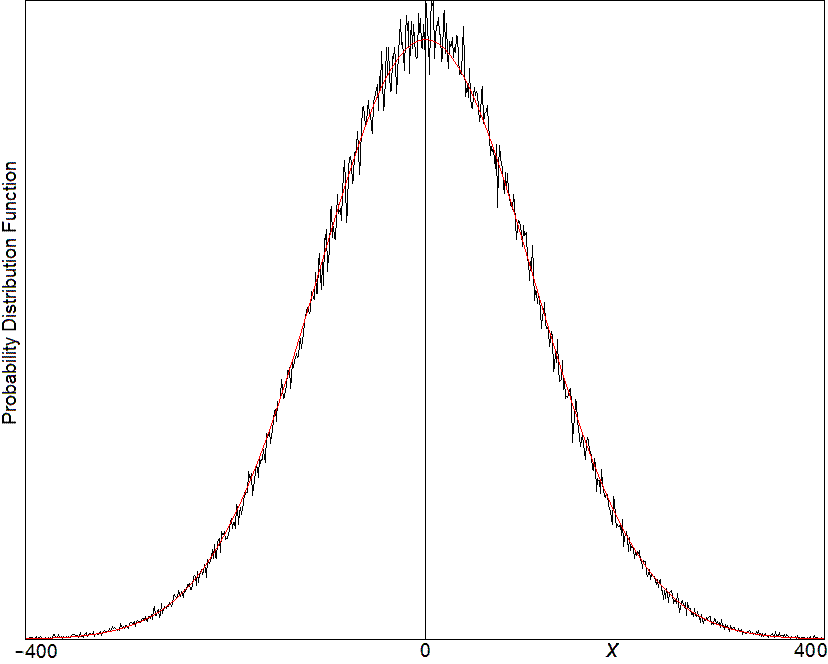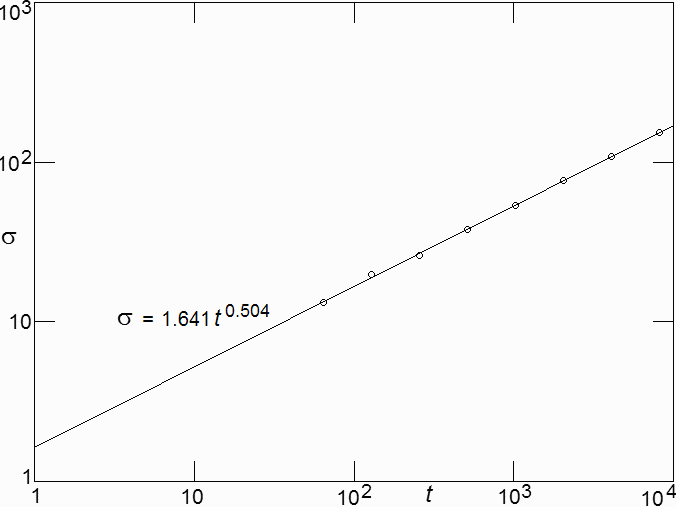# A Simple Chaotic Delay Differential Equation

J. C. Sprott

Received 21 November 2006; received in revised form 16 January 2007; accepted 18 January 2007

### ABSTRACT

The simplest chaotic delay differential equation with a sinusoidal nonlinearity is described, including the route to chaos, Lyapunov exponent spectrum, and chaotic diffusion. It is prototypical of many other high-dimensional chaotic systems.

Ref: J. C. Sprott, Phys. Lett. A 366, 397-402 (2007)

The complete paper is available in PDF format.

Fig. 1. Variation of Lyapunov exponents, Kaplan-Yorke dimension, Kolmogorov-Sinai entropy, and local maximum of x with time delay tau.Fig. 2. Plots of x(t) versus x(t - tau) for increasing tau showing the growth of the limit cycle, period doubling, and the onset of chaos at tau ~ 5.Fig. 3. Return maps (modulo 2pi) for increasing tau showing the increasing complexity of the attractor beyond the onset of chaos at tau ~ 5.Fig. 4. Time history of x, xtau, and dx/dt for tau = 20 showing Brownian motion.Fig. 5. Probability distribution function of x for 5 × 105 initial conditions near the origin after a time of 4 × 103 with tau = 20. The red curve is a Gaussian distribution with the same standard deviation and area.Fig. 6. Standard deviation of 5 × 105 trajectories starting near the origin versus time for tau = 20.